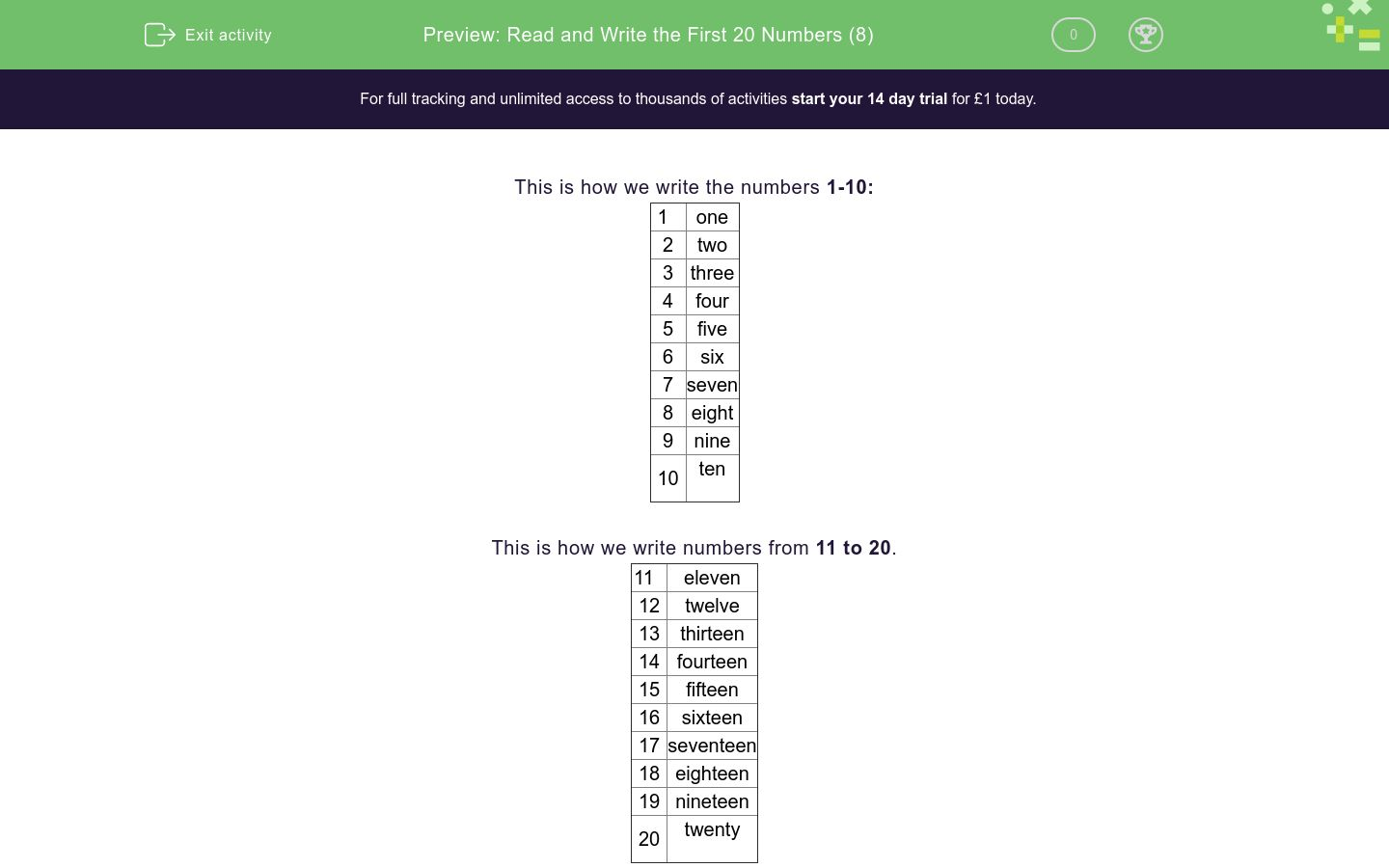# Read and Write the First 20 Numbers (8)

In this worksheet, children must read numbers written in numerals and write the number in words.Key stage:  KS 1

Curriculum topic:   Number: Number and Place Value

Curriculum subtopic:   Read and Write Number Words to 20

Difficulty level:### QUESTION 1 of 10

This is how we write the numbers 1-10:

 1 one 2 two 3 three 4 four 5 five 6 six 7 seven 8 eight 9 nine 10 ten

This is how we write numbers from 11 to 20.

 11 eleven 12 twelve 13 thirteen 14 fourteen 15 fifteen 16 sixteen 17 seventeen 18 eighteen 19 nineteen 20 twenty

Write this number using a word:

3

Write this number using a word:

13

Write this number using a word:

14

Write this number using a word:

8

Write this number using a word:

10

Write this number using a word:

17

Write this number using a word:

19

Write this number using a word:

5

Write this number using a word:

18

Write this number using a word:

11

• Question 1

Write this number using a word:

3

three
Three
EDDIE SAYS
Three is 3.
• Question 2

Write this number using a word:

13

thirteen
Thirteen
EDDIE SAYS
Thirteen is 13.
• Question 3

Write this number using a word:

14

fourteen
Fourteen
EDDIE SAYS
Fourteen is 14.
• Question 4

Write this number using a word:

8

eight
Eight
EDDIE SAYS
Eight is 8.
• Question 5

Write this number using a word:

10

ten
Ten
EDDIE SAYS
Ten is 10.
• Question 6

Write this number using a word:

17

seventeen
Seventeen
EDDIE SAYS
Seventeen is 17.
• Question 7

Write this number using a word:

19

nineteen
Nineteen
EDDIE SAYS
Nineteen is 19.
• Question 8

Write this number using a word:

5

five
Five
EDDIE SAYS
Five is 5.
• Question 9

Write this number using a word:

18

eighteen
Eighteen
EDDIE SAYS
Eighteen is 18.
• Question 10

Write this number using a word:

11

eleven
Eleven
EDDIE SAYS
Eleven is 11.
---- OR ----